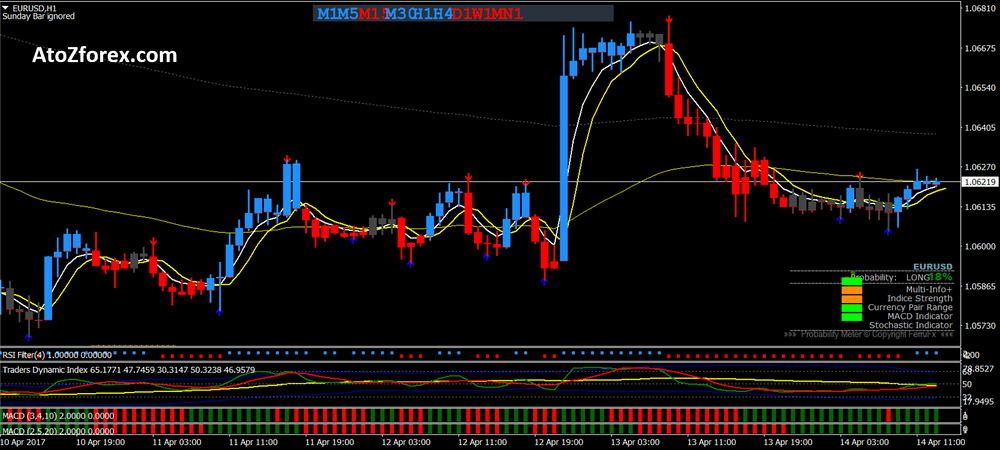Forex King trading system is a part of the MT4 trading system that uses a trend-momentum system based on moving averages TDI, MACD, and RSI. This system for trading with bars, candle chart and Renko chart.

The difference is that with Renko the trend chart can be followed even without reference to the slow average of 200 periods. The Forex King trading system works on every currency pair and we recommend using it for 15 min or higher time frame.

Forex King trading system used a strategy based on the indicators which signify buying and selling signal. Metatrader Indicators for Forex King trading system are as follows:

• Exponential Moving Average 4 period
• Exponential Moving Average 5 period
• Exponential Moving Average 200 period
• Exponential Moving Average 60 period
• MACD Candle (2,5, 20)
• Auto pivot levels
• Probability Meter: MACD 5,13, 1 – Stochastic 20, 2, 2,
• RSI Filter 2 period (with Renko chart) 4 period with (candle e bar chart),
• TDI (RSI 13, Volatility band 34);
• MACD bars 3, 4, 10.
• MACD bars 2, 5, 10Buying signal: Buy when the Price moves above MA 60 and 200 with Renko chart price above 60 and consider the parameters as follows:

• MA 4>MA5>MA60>MA200.
• TDI red line above the green and yellow line.
• RSI blue bar.
• MACD Candle blue bar.
• MACD red bar.
• Probability meter > 55 <90 optional.

Selling signal:  Sell when the Price moves below MA 60 and 200 with Renko chart price above 60 and consider the parameters as follows:

• MA 4<MA5<MA60<MA200.
• RSI red bar.
• TDI red line below green and yellow line
• MACD Candle red bar.
• MACD red bar.
• Probability meter < 55 >10 optional.